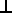# Maths - Projections of lines on planes

This was my original working before Xavier pointed out the inconsistencies. (back to main page)

## Alternative using Matrix representation

#### perpendicular component

AB = (AB) * B/|B|2

AB can be calculated as follows:

x = Ay * Bz - By * Az
y = Az * Bx - Bz * Ax
z = Ax * By - Bx * Ay

So,

(AB)x = ((Az * Bx - Bz * Ax) * Bz - By * (Ax * By - Bx * Ay)) / (Bx2 + By2 + Bz2)
(AB)y = ((Ax * By - Bx * Ay) * Bx - Bz * (Ay * Bz - By * Az)) / (Bx2 + By2 + Bz2)
(AB)z = ((Ay * Bz - By * Az) * By - Bx * (Az * Bx - Bz * Ax)) / (Bx2 + By2 + Bz2)

grouping terms,

(AB)x = (Az * Bx* Bz - Bz * Ax* Bz - Ax * By* By + Bx * Ay* By) / (Bx2 + By2 + Bz2)
(AB)y = (Ax * By* Bx - Bx * Ay* Bx - Ay * Bz*Bz + By * Az*Bz) / (Bx2 + By2 + Bz2)
(AB)z = (Ay * Bz* By - By * Az* By - Az * Bx*Bx + Bz * Ax*Bx) / (Bx2 + By2 + Bz2)

In matrix form,

(AB) = 1 / (Bx2 + By2 + Bz2)*
 -Bz* Bz- By* By Bx * By Bx* Bz By* Bx -Bx*Bx- Bz*Bz By *Bz Bz *Bx Bz* By -By*By- Bx*Bx
[A]

I think the signs of all terms should be inverted, see error heading below, can anyone help?

### Alternative Form

As pointed out here, If B is normalised (unit length) then Bx2 + By2 + Bz2 =1 so we get:

(AB) =
 Bx*Bx - 1 Bx * By Bx* Bz By* Bx By*By- 1 By *Bz Bz *Bx Bz* By Bz*Bz - 1
[A]

which is:

(AB) = (
 Bx By Bz
*
 Bx By Bz
-[I])[A]

which is:

(AB) = (B * Bt - [I]) [A]

where:

• [I] = unit matrix
• Bt = transpose of B vector

As this is quite a simple equation I wonder if there is a simpler way to derive it? perhaps the method of least squares discussed here.

#### parallel component

A || P = A • P * P/|B|2

A • P can be calculated as follows:

Ax * Bx + Ay * By + Az * Bz

so,

(A || P)x = (Ax * Bx + Ay * By + Az * Bz) * Bx / (Bx2 + By2 + Bz2)
(A || P)y = (Ax * Bx + Ay * By + Az * Bz) * By/ (Bx2 + By2 + Bz2)
(A || P)z = (Ax * Bx + Ay * By + Az * Bz) * Bz/ (Bx2 + By2 + Bz2)

in matrix form this is:

(A || P) = 1 / (Bx2 + By2 + Bz2)*
 Bx2 Bx * By Bx * Bz By * Bx By2 By * Bz Bz * Bx Bz * By Bz2
[A]

### Alternative Form

If B is normalised (unit length) then Bx2 + By2 + Bz2 =1 so we get:

(A || P) =
 Bx * Bx Bx * By Bx * Bz By * Bx By * By By * Bz Bz * Bx Bz * By Bz * Bz
[A]

which is:

(AP) = (
 Bx By Bz
*
 Bx By Bz
)[A]

which is:

(AP) = (B * Bt) [A]

where:

• Bt = transpose of B vector

As this is quite a simple equation I wonder if there is a simpler way to derive it? perhaps the method of least squares discussed here.

## Error

we have shown above that:

A = A || B + AB

which would give:

A = (B * Bt - [I] + B * Bt)[A]

which should simplify to A = [I][A] but it does not so it looks like the sign of (AB) is inverted can anyone see where I went wrong?New Foundations for Classical Mechanics (Fundamental Theories of Physics). This is very good on the geometric interpretation of this algebra. It has lots of insights into the mechanics of solid bodies. I still cant work out if the position, velocity, etc. of solid bodies can be represented by a 3D multivector or if 4 or 5D multivectors are required to represent translation and rotation.